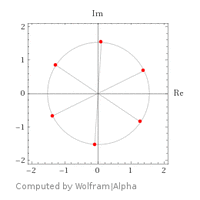# Systemic Nth roots of a number

#### pka

##### Elite Member
There are six sixth roots of $$\displaystyle -12+5i$$ see here.See that there are six of them. Each of them is on a circle centered at the origin of radius $$\displaystyle |-12+5i|^{1/6}=\large\sqrt{13}$$
However, the salient feature is the fact the the are evenly spaced on the circle and the central angle between any to adjacent is $$\displaystyle \frac{2\pi}{6}=\frac{\pi}{3}$$. see here
Here is a step by step outline: If $$\displaystyle N>1$$ is a positive integer and $$\displaystyle z=x+yi$$ is a complex number in which $$\displaystyle x\cdot y\ne 0$$ (if that case fails there are special considerations).
Because all of this necessitates $$\displaystyle z$$ being in polar form, I digress into this:
$$\displaystyle \arg(z)=\arg(x + yi) = \left\{ {\begin{array}{{rl}} {\arctan \left( {\dfrac{y}{x}} \right),}&{x > 0} \\ {\arctan \left( {\dfrac{y}{x}} \right) + \pi ,}&{x < 0\;\& \;y > 0} \\ {\arctan \left( {\dfrac{y}{x}} \right) - \pi ,}&{x < 0\;\& \;y < 0} \end{array}} \right.$$ _______keep in mind that $$\displaystyle x\cdot y\ne 0 \text{ implies }x\ne 0~\&~y\ne 0$$.

Return to the job at hand. If $$\displaystyle z=|z|\exp(\theta i)$$ where $$\displaystyle \theta=\arg(z)$$
Define $$\displaystyle \zeta={\large\sqrt[N]{|z|}}\exp\left(\frac{\theta i}{N}\right)$$ _____Do you see that $$\displaystyle \zeta^N=z~?$$ I certainly hope you do !
Now we need a "rotator " to distribute the roots of $$\displaystyle z$$ equality spaced around a circle. $$\displaystyle \rho=\exp\left(\frac{2\pi i}{N}\right)$$
The set $$\displaystyle \left\{\zeta\cdot\rho^k|k=0,1,\cdots N-1 \right\}$$ is the collection of the $$\displaystyle N^{th}$$ roots of $$\displaystyle z$$.

Here is a practice question: List the seven seventh roots of $$\displaystyle -6-8i$$.

•topsquark